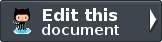# Introduction to Zend\Math¶

Zend\Math namespace provides general mathematical functions. So far the supported functionalities are:

• Zend\Math\Rand, a random number generator;
• Zend\Math\BigInteger, a library to manage big integers.

We expect to add more functionalities in the future.

## Random number generator¶

Zend\Math\Rand implements a random number generator that is able to generate random numbers for general purpose usage and for cryptographic scopes. To generate good random numbers this component uses the OpenSSL and the Mcrypt extension of PHP. If you don’t have the OpenSSL or the Mcrypt extension installed in your environment the component will use the mt_rand function of PHP as fallback. The mt_rand is not considered secure for cryptographic purpose, that means if you will try to use it to generate secure random number the class will throw an exception.

In particular, the algorithm that generates random bytes in Zend\Math\Rand tries to call the openssl_random_pseudo_bytes function of the OpenSSL extension if installed. If the OpenSSL extension is not present in the system the algorithm tries to use the the mcrypt_create_iv function of the Mcrypt extension (using the MCRYPT_DEV_URANDOM parameter). Finally, if the OpenSSL and Mcrypt are not installed the generator uses the mt_rand function of PHP.

The Zend\Math\Rand class offers the following methods to generate random values:

• getBytes(\$length, \$strong = false) to generate a random set of \$length bytes;
• getBoolean(\$strong = false) to generate a random boolean value (true or false);
• getInteger(\$min, \$max, \$strong = false) to generate a random integer between \$min and \$max;
• getFloat(\$strong = false) to generate a random float number between 0 and 1;
• getString(\$length, \$charlist = null, \$strong = false) to generate a random string of \$length characters using the alphabet \$charlist (if not provided the default alphabet is the Base64).

In all these methods the parameter \$strong specify the usage of a strong random number generator. We suggest to set the \$strong to true if you need to generate random number for cryptographic and security implementation.

If \$strong is set to true and you try to generate random values in a PHP environment without the OpenSSL and the Mcrypt extensions the component will throw an Exception.

Below we reported an example on how to generate random data using Zend\Math\Rand.

 ``` 1 2 3 4 5 6 7 8 9 10 11 12 13 14 15 16``` ```use Zend\Math\Rand; \$bytes = Rand::getBytes(32, true); printf("Random bytes (in Base64): %s\n", base64_encode(\$bytes)); \$boolean = Rand::getBoolean(); printf("Random boolean: %s\n", \$boolean ? 'true' : 'false'); \$integer = Rand::getInteger(0,1000); printf("Random integer in [0-1000]: %d\n", \$integer); \$float = Rand::getFloat(); printf("Random float in [0-1): %f\n", \$float); \$string = Rand::getString(32, 'abcdefghijklmnopqrstuvwxyz', true); printf("Random string in latin alphabet: %s\n", \$string); ```

## Big integers¶

Zend\Math\BigInteger\BigInteger offers a class to manage arbitrary length integer. PHP supports integer numbers with a maximum value of PHP_INT_MAX. If you need to manage integers bigger than PHP_INT_MAX you have to use external libraries or PHP extensions like GMP or BC Math.

Zend\Math\BigInteger\BigInteger is able to manage big integers using the GMP or the BC Math extensions as adapters.

The mathematical functions implemented in Zend\Math\BigInteger\BigInteger are:

• sub(\$leftOperand, \$rightOperand), subtract two big integers;
• mul(\$leftOperand, \$rightOperand), multiply two big integers;
• div(\$leftOperand, \$rightOperand), divide two big integers (this method returns only integer part of result);
• pow(\$operand, \$exp), raise a big integers to another;
• sqrt(\$operand), get the square root of a big integer;
• abs(\$operand), get the absolute value of a big integer;
• mod(\$leftOperand, \$modulus), get modulus of a big integer;
• powmod(\$leftOperand, \$rightOperand, \$modulus), raise a big integer to another, reduced by a specified modulus;
• comp(\$leftOperand, \$rightOperand), compare two big integers, returns < 0 if leftOperand is less than rightOperand; > 0 if leftOperand is greater than rightOperand, and 0 if they are equal;
• intToBin(\$int, \$twoc = false), convert big integer into it’s binary number representation;
• binToInt(\$bytes, \$twoc = false), convert binary number into big integer;
• baseConvert(\$operand, \$fromBase, \$toBase = 10), convert a number between arbitrary bases;

Below is reported an example using the BC Math adapter to calculate the sum of two integer random numbers with 100 digits.

 ``` 1 2 3 4 5 6 7 8 9 10 11 12``` ```use Zend\Math\BigInteger\BigInteger; use Zend\Math\Rand; \$bigInt = BigInteger::factory('bcmath'); \$x = Rand::getString(100,'0123456789'); \$y = Rand::getString(100,'0123456789'); \$sum = \$bigInt->add(\$x, \$y); \$len = strlen(\$sum); printf("%{\$len}s +\n%{\$len}s =\n%s\n%s\n", \$x, \$y, str_repeat('-', \$len), \$sum); ```

As you can see in the code the big integers are managed using strings. Even the result of the sum is represented as a string.

Below is reported another example using the BC Math adapter to generate the binary representation of a negative big integer of 100 digits.

 ``` 1 2 3 4 5 6 7 8 9 10 11 12 13 14 15 16 17 18 19``` ```use Zend\Math\BigInteger\BigInteger; use Zend\Math\Rand; \$bigInt = BigInteger::factory('bcmath'); \$digit = 100; \$x = '-' . Rand::getString(\$digit,'0123456789'); \$byte = \$bigInt->intToBin(\$x); printf("The binary representation of the big integer with \$digit digit:\n%s\nis (in Base64 format): %s\n", \$x, base64_encode(\$byte)); printf("Length in bytes: %d\n", strlen(\$byte)); \$byte = \$bigInt->intToBin(\$x, true); printf("The two's complement binary representation of the big integer with \$digit digit:\n%s\nis (in Base64 format): %s\n", \$x, base64_encode(\$byte)); printf("Length in bytes: %d\n", strlen(\$byte)); ```

We generated the binary representation of the big integer number using the default binary format and the two’s complement representation (specified with the true parameter in the intToBin function).

#### Previous topic

Zend\Mail\Transport\FileOptions

#### Next topic

Overview

Note: You need to stay logged into your GitHub account to contribute to the documentation.### Edit this document

The source code of this file is hosted on GitHub. Everyone can update and fix errors in this document with few clicks - no downloads needed.